Courses

# Quant Mock Test - 15

## 15 Questions MCQ Test Mock Test Series for CLAT 2020 | Quant Mock Test - 15

Description
This mock test of Quant Mock Test - 15 for CLAT helps you for every CLAT entrance exam. This contains 15 Multiple Choice Questions for CLAT Quant Mock Test - 15 (mcq) to study with solutions a complete question bank. The solved questions answers in this Quant Mock Test - 15 quiz give you a good mix of easy questions and tough questions. CLAT students definitely take this Quant Mock Test - 15 exercise for a better result in the exam. You can find other Quant Mock Test - 15 extra questions, long questions & short questions for CLAT on EduRev as well by searching above.
QUESTION: 1

### Directions: Study the following table carefully and answer the questions given below. Number of questions asked from different subjects in the following years in a Bank Competition Exam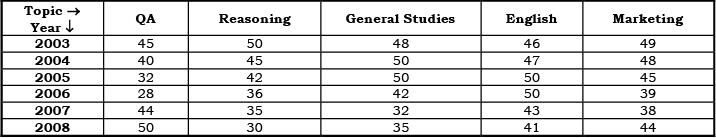Q. The average number of questions asked from which subject is the maximum?

Solution: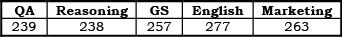Note: After a simple comparison of the no. of questions asked in English with other topics, we find that it is maximum for English.

QUESTION: 2

### Directions: Study the following table carefully and answer the questions given below. Number of questions asked from different subjects in the following years in a Bank Competition ExamQ. The average number of questions asked from QA and Reasoning in 2003 is not equal to the average number of questions asked from which of the following?

Solution:

For “a” → 50 + 45 = 95
“b” → 50 + 45 = 95. “c” → 50 + 45 = 95
“d” → 50 + 32 = 82

QUESTION: 3

### Directions: Study the following table carefully and answer the questions given below. Number of questions asked from different subjects in the following years in a Bank Competition ExamQ. The percentage of questions asked in Reasoning is the maximum among the given subjects in the year?

Solution:

Only in 2003, the required percentage is more than 20%.

QUESTION: 4

Directions: Study the following table carefully and answer the questions given below.
Number of questions asked from different subjects in the following years in a Bank Competition ExamQ. The average number of questions asked in the given years from General Studies is approximately equal to

Solution:

257/6 = 42.8 ≈ 43

QUESTION: 5

Directions: Study the following table carefully and answer the questions given below.
Number of questions asked from different subjects in the following years in a Bank Competition ExamQ. The percentage decrease in the number of questions asked in 2007 from Reasoning as compared with previous year is equal to.

Solution:

Reqd % decrease =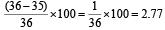QUESTION: 6

Directions: Answer the following questions on the basis of the information given below:
India’s foreign exchange reserves (in Rs crore)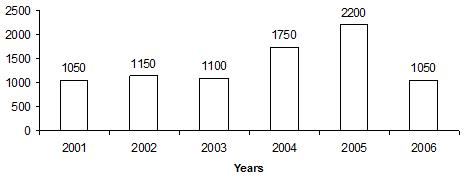Q. The foreign exchange reserves in 2004 is approximately what percent of that in 2002?

Solution:

1750 / 1150 x 100 ≈ 150%

QUESTION: 7

Directions: Answer the following questions on the basis of the information given below:
India’s foreign exchange reserves (in Rs crore)Q. In how many years is the foreign exchange greater than the average figure over these years?

Solution:

Average ≈ 1383 (2/3)
So, in yrs 2004 & 2005 the given value is more than the average value.

QUESTION: 8

Directions: Answer the following questions on the basis of the information given below:
India’s foreign exchange reserves (in Rs crore)Q. The increase in foreign exchange was approximately 10% of that in the previous year, in which of the following years?

Solution:

Only in year 2002

QUESTION: 9

Directions: Answer the following questions on the basis of the information given below:
India’s foreign exchange reserves (in Rs crore)Q. In which year is the percentage increase in foreign exchange over the previous year the maximum?

Solution:
QUESTION: 10

Directions: Answer the following questions on the basis of the information given below:
India’s foreign exchange reserves (in Rs crore)Q. What is the approximate percentage decline in the foreign exchange in 2006?

Solution:

2200 – 1050 / 2200 x 100 ≈ 50%

QUESTION: 11

Directions: Study the following graph carefully to answer these questions.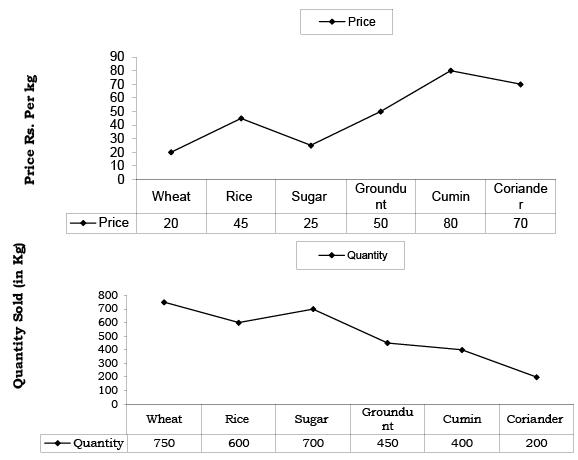Q. What is the average price per kg of wheat and rice sold?

Solution: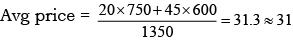QUESTION: 12

Directions: Study the following graph carefully to answer these questions.Q. If cumin is sold at a 10% discount, the quantity sold doubles. What will be the cost of the total quantity of Cumin sold on discount?

Solution: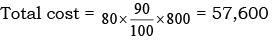QUESTION: 13

Directions: Study the following graph carefully to answer these questions.Q. Out of the total quantity of sugar sold, half the quantity is sold at the given rate while half the quantity is sold on a price increased by 20%. What is the total cost of the entire quantity of sugar sold?

Solution:

Total cost = 350 x 25 + 350 x 6/5 x 25 = 19250

QUESTION: 14

Directions: Study the following graph carefully to answer these questions.Q. If the quantities sold of groundnut and cumin are interchanged, what will be the total cost of the quantities sold for these two items together?

Solution:

Total cost = 50 x 400 + 80 x 450 = 56,000

QUESTION: 15

Directions: Study the following graph carefully to answer these questions.Q. If the quantity of coriander sold is increased by 200% and the price per kg is reduced by 8%, what will be the total cost of coriander sold?

Solution:

The correct option is C.

Original quantity of coriander sold = 200kg

New quantity if it is increased by 200% = 200+200/100*200 = 600kg

Original price per kg = Rs 70

New price if it is reduced by 8% = 70-8/100*70 = 64.60

Total cost = 600*64.6

= 38,640